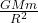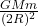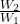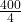## A weight watcher who normally weighs 400 N stands on top of a very tall ladder so she is one Earth radius above the Earth’s surface. How muc

Question

A weight watcher who normally weighs 400 N stands on top of a very tall ladder so she is one Earth radius above the Earth’s surface. How much would she weigh there

in progress 0
3 weeks 2021-08-31T14:56:08+00:00 1 Answers 0 views 0

1. ## Her weight at the height of R will be 100 N

Explanation:

The weight of the body on the surface of earth can be found by the relation

W₁ =I

Here G is gravitational constant and M is the mass of earth .

m is the mass of the body

and R is the radius of earth  or the distance of body from the center of the earth .

Now  she moves to height equal to R . Thus the distance from center of earth becomes 2 R .

Thus Weight now is W₂ =II

Dividing II by I , we have=But W₁ = 400 N

Thus W₂ == 100 N